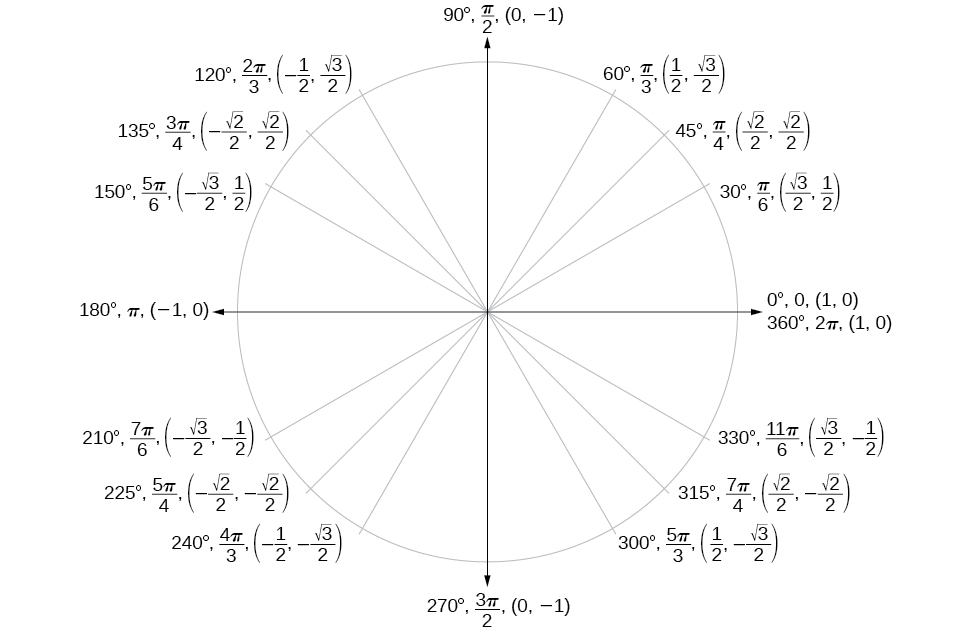# 7.3 Unit circle  (Page 6/11)

 Page 6 / 11

## Using reference angles to find sine and cosine

1. Using a reference angle, find the exact value of $\text{\hspace{0.17em}}\mathrm{cos}\left(150°\right)\text{\hspace{0.17em}}$ and $\text{\hspace{0.17em}}\text{sin}\left(150°\right).$
2. Using the reference angle, find $\text{\hspace{0.17em}}\mathrm{cos}\text{\hspace{0.17em}}\frac{5\pi }{4}\text{\hspace{0.17em}}$ and $\text{\hspace{0.17em}}\mathrm{sin}\text{\hspace{0.17em}}\frac{5\pi }{4}.$
1. $150°\text{\hspace{0.17em}}$ is located in the second quadrant. The angle it makes with the x -axis is $\text{\hspace{0.17em}}180°-150°=30°,$ so the reference angle is $\text{\hspace{0.17em}}30°.$

This tells us that $\text{\hspace{0.17em}}150°\text{\hspace{0.17em}}$ has the same sine and cosine values as $\text{\hspace{0.17em}}30°,$ except for the sign.

$\begin{array}{ccc}\mathrm{cos}\left(30°\right)=\frac{\sqrt{3}}{2}& \phantom{\rule{1em}{0ex}}\text{and}\phantom{\rule{1em}{0ex}}& \mathrm{sin}\left(30°\right)=\frac{1}{2}\end{array}$

Since $\text{\hspace{0.17em}}150°\text{\hspace{0.17em}}$ is in the second quadrant, the x -coordinate of the point on the circle is negative, so the cosine value is negative. The y -coordinate is positive, so the sine value is positive.

$\begin{array}{ccc}\mathrm{cos}\left(150°\right)=\frac{\sqrt{3}}{2}& \phantom{\rule{1em}{0ex}}\text{and}\phantom{\rule{1em}{0ex}}& \mathrm{sin}\left(150°\right)=\frac{1}{2}\end{array}$
2. $\frac{5\pi }{4}\text{\hspace{0.17em}}$ is in the third quadrant. Its reference angle is $\text{\hspace{0.17em}}\frac{5\pi }{4}-\pi =\frac{\pi }{4}.\text{\hspace{0.17em}}$ The cosine and sine of $\text{\hspace{0.17em}}\frac{\pi }{4}\text{\hspace{0.17em}}$ are both $\text{\hspace{0.17em}}\frac{\sqrt{2}}{2}.\text{\hspace{0.17em}}$ In the third quadrant, both $\text{\hspace{0.17em}}x\text{\hspace{0.17em}}$ and $\text{\hspace{0.17em}}y\text{\hspace{0.17em}}$ are negative, so:
$\begin{array}{ccc}\mathrm{cos}\phantom{\rule{0.03em}{0ex}}\frac{5\pi }{4}=-\frac{\sqrt{2}}{2}& \phantom{\rule{1em}{0ex}}\text{and}\phantom{\rule{1em}{0ex}}& \mathrm{sin}\phantom{\rule{0.03em}{0ex}}\frac{5\pi }{4}=-\frac{\sqrt{2}}{2}\end{array}$
1. Use the reference angle of $\text{\hspace{0.17em}}315°\text{\hspace{0.17em}}$ to find $\text{\hspace{0.17em}}\mathrm{cos}\left(315°\right)\text{\hspace{0.17em}}$ and $\text{\hspace{0.17em}}\mathrm{sin}\left(315°\right).$
2. Use the reference angle of $\text{\hspace{0.17em}}-\frac{\pi }{6}\text{\hspace{0.17em}}$ to find $\text{\hspace{0.17em}}\mathrm{cos}\left(-\frac{\pi }{6}\right)\text{\hspace{0.17em}}$ and $\text{\hspace{0.17em}}\mathrm{sin}\left(-\frac{\pi }{6}\right).$
1. $\text{cos}\left(-\frac{\pi }{6}\right)=\frac{\sqrt{3}}{2},\mathrm{sin}\left(-\frac{\pi }{6}\right)=-\frac{1}{2}$

## Using reference angles to find coordinates

Now that we have learned how to find the cosine and sine values for special angles in the first quadrant, we can use symmetry and reference angles to fill in cosine and sine values for the rest of the special angles on the unit circle    . They are shown in [link] . Take time to learn the $\text{\hspace{0.17em}}\left(x,y\right)\text{\hspace{0.17em}}$ coordinates of all of the major angles in the first quadrant.Special angles and coordinates of corresponding points on the unit circle

In addition to learning the values for special angles, we can use reference angles to find $\text{\hspace{0.17em}}\left(x,y\right)\text{\hspace{0.17em}}$ coordinates of any point on the unit circle, using what we know of reference angles along with the identities

First we find the reference angle corresponding to the given angle. Then we take the sine and cosine values of the reference angle, and give them the signs corresponding to the y - and x -values of the quadrant.

Given the angle of a point on a circle and the radius of the circle, find the $\text{\hspace{0.17em}}\left(x,y\right)\text{\hspace{0.17em}}$ coordinates of the point.

1. Find the reference angle by measuring the smallest angle to the x -axis.
2. Find the cosine and sine of the reference angle.
3. Determine the appropriate signs for $\text{\hspace{0.17em}}x\text{\hspace{0.17em}}$ and $\text{\hspace{0.17em}}y\text{\hspace{0.17em}}$ in the given quadrant.

## Using the unit circle to find coordinates

Find the coordinates of the point on the unit circle at an angle of $\text{\hspace{0.17em}}\frac{7\pi }{6}.$

We know that the angle $\text{\hspace{0.17em}}\frac{7\pi }{6}\text{\hspace{0.17em}}$ is in the third quadrant.

First, let’s find the reference angle by measuring the angle to the x -axis. To find the reference angle of an angle whose terminal side is in quadrant III, we find the difference of the angle and $\text{\hspace{0.17em}}\pi .$

$\frac{7\pi }{6}-\pi =\frac{\pi }{6}$

Next, we will find the cosine and sine of the reference angle.

$\begin{array}{cc}\mathrm{cos}\left(\frac{\pi }{6}\right)=\frac{\sqrt{3}}{2}\hfill & \phantom{\rule{1em}{0ex}}\mathrm{sin}\left(\frac{\pi }{6}\right)=\frac{1}{2}\hfill \end{array}$

We must determine the appropriate signs for x and y in the given quadrant. Because our original angle is in the third quadrant, where both $\text{\hspace{0.17em}}x\text{\hspace{0.17em}}$ and $\text{\hspace{0.17em}}y\text{\hspace{0.17em}}$ are negative, both cosine and sine are negative.

$\begin{array}{ccc}\hfill \mathrm{cos}\left(\frac{7\pi }{6}\right)& =& -\frac{\sqrt{3}}{2}\hfill \\ \hfill \mathrm{sin}\left(7\pi \right)& =& -\frac{1}{2}\hfill \end{array}$

Now we can calculate the $\text{\hspace{0.17em}}\left(x,y\right)\text{\hspace{0.17em}}$ coordinates using the identities $\text{\hspace{0.17em}}x=\mathrm{cos}\text{\hspace{0.17em}}\theta \text{\hspace{0.17em}}$ and $\text{\hspace{0.17em}}y=\mathrm{sin}\text{\hspace{0.17em}}\theta .$

The coordinates of the point are $\text{\hspace{0.17em}}\left(-\frac{\sqrt{3}}{2},-\frac{1}{2}\right)\text{\hspace{0.17em}}$ on the unit circle.

Find the coordinates of the point on the unit circle at an angle of $\text{\hspace{0.17em}}\frac{5\pi }{3}.$

$\left(\frac{1}{2},-\frac{\sqrt{3}}{2}\right)$

#### Questions & Answers

write down the polynomial function with root 1/3,2,-3 with solution
if A and B are subspaces of V prove that (A+B)/B=A/(A-B)
write down the value of each of the following in surd form a)cos(-65°) b)sin(-180°)c)tan(225°)d)tan(135°)
Prove that (sinA/1-cosA - 1-cosA/sinA) (cosA/1-sinA - 1-sinA/cosA) = 4
what is the answer to dividing negative index
In a triangle ABC prove that. (b+c)cosA+(c+a)cosB+(a+b)cisC=a+b+c.
give me the waec 2019 questions
the polar co-ordinate of the point (-1, -1)
prove the identites sin x ( 1+ tan x )+ cos x ( 1+ cot x )= sec x + cosec x
tanh`(x-iy) =A+iB, find A and B
B=Ai-itan(hx-hiy)
Rukmini
what is the addition of 101011 with 101010
If those numbers are binary, it's 1010101. If they are base 10, it's 202021.
Jack
extra power 4 minus 5 x cube + 7 x square minus 5 x + 1 equal to zero
the gradient function of a curve is 2x+4 and the curve passes through point (1,4) find the equation of the curve
1+cos²A/cos²A=2cosec²A-1
test for convergence the series 1+x/2+2!/9x3ByByByByBy